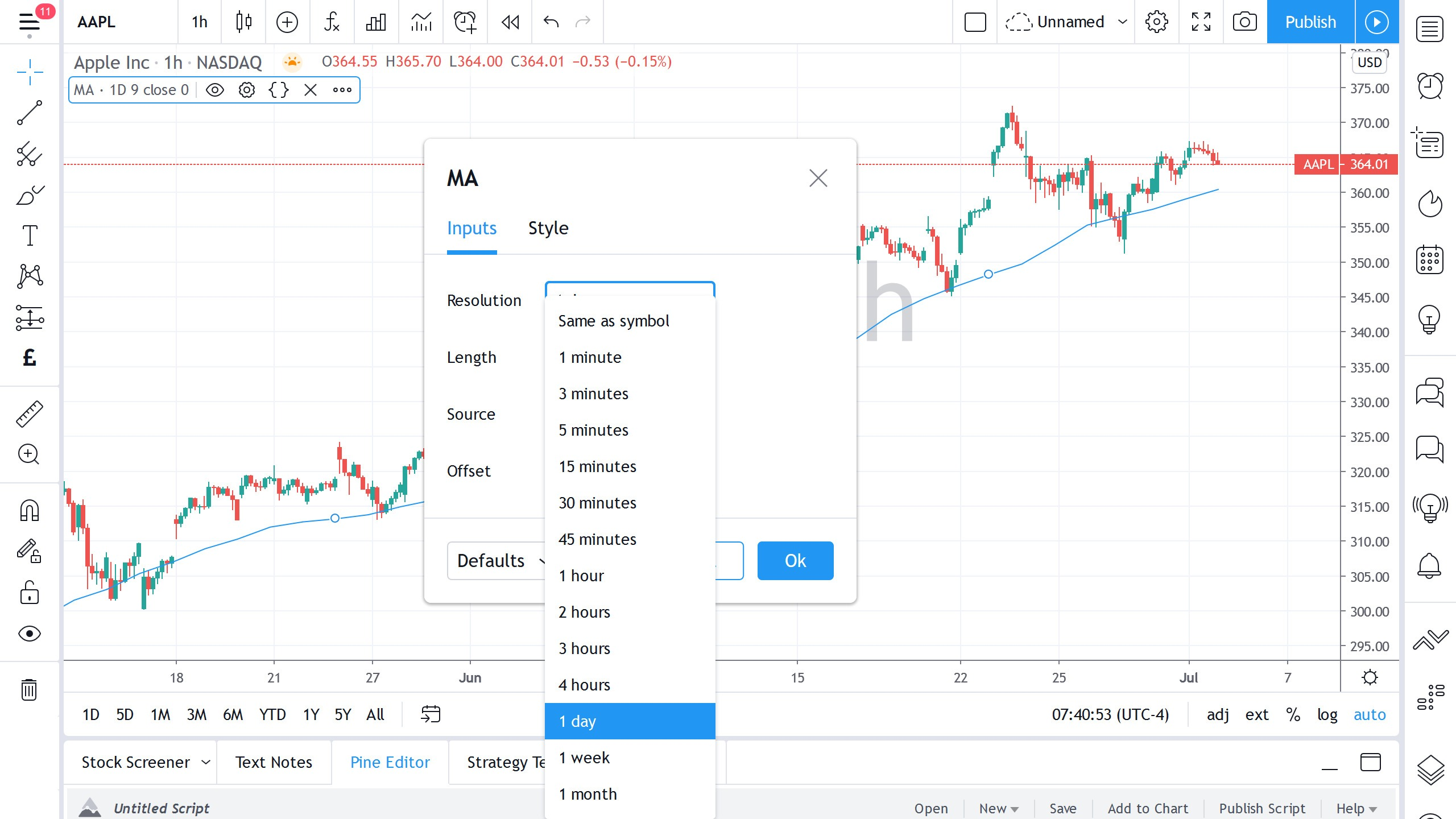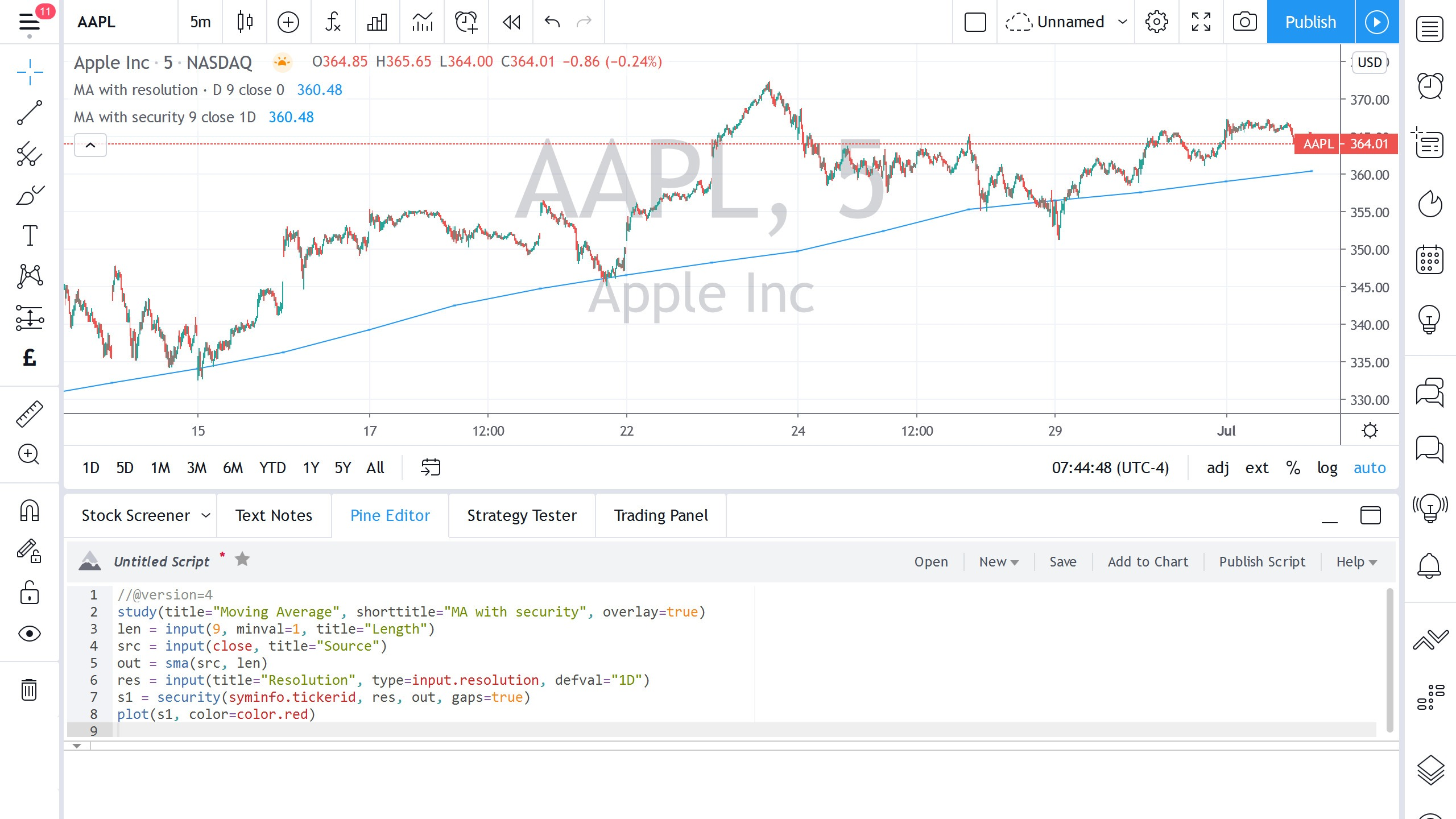# 如何使用MTF功能？Pine编程人员可以在自己的脚本中使用与内建指标中相同的时间周期参数。通过将其简单地增加到脚本的study声明语句中，编程人员现在可以轻松地将MTF功能添加到脚本中，并让用户确定希望指标运行的时间周期。

``````//@version=4
study(title="Moving Average", shorttitle="MA with security", overlay=true)
len = input(9, minval=1, title="Length")
src = input(close, title="Source")
out = sma(src, len)
res = input(title="Resolution", type=input.resolution, defval="1D")
s1 = security(syminfo.tickerid, res, out, gaps=true)
plot(s1, color=color.red)``````
Java

``````//@version=4study(title="Moving Average", shorttitle="MA with resolution", overlay=true, resolution="D")
len = input(9, minval=1, title="Length")
src = input(close, title="Source")
offset = input(title="Offset", type=input.integer, defval=0, minval=-500, maxval=500)
out = sma(src, len)
plot(out, color=color.blue, title="MA", offset=offset)``````
Java